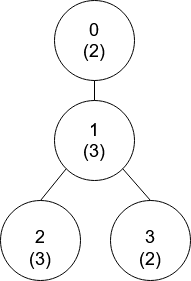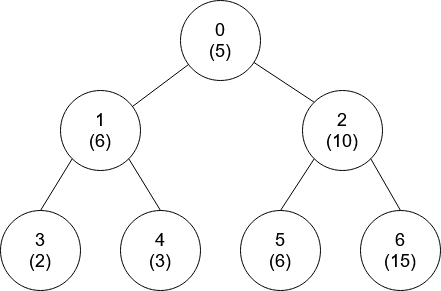# 互质树

## 1766. 互质树 (Hard)```输入：nums = [2,3,3,2], edges = [[0,1],[1,2],[1,3]]

- 节点 0 没有互质祖先。
- 节点 1 只有一个祖先节点 0 。它们的值是互质的（gcd(2,3) == 1）。
- 节点 2 有两个祖先节点，分别是节点 1 和节点 0 。节点 1 的值与它的值不是互质的（gcd(3,3) == 3）但节点 0 的值是互质的(gcd(2,3) == 1)，所以节点 0 是最近的符合要求的祖先节点。
- 节点 3 有两个祖先节点，分别是节点 1 和节点 0 。它与节点 1 互质（gcd(3,2) == 1），所以节点 1 是离它最近的符合要求的祖先节点。
``````输入：nums = [5,6,10,2,3,6,15], edges = [[0,1],[0,2],[1,3],[1,4],[2,5],[2,6]]

```

• `nums.length == n`
• `1 <= nums[i] <= 50`
• `1 <= n <= 105`
• `edges.length == n - 1`
• `edges[j].length == 2`
• `0 <= uj, vj < n`
• `uj != vj`

### 相关话题

[] [深度优先搜索] [广度优先搜索] [数学]